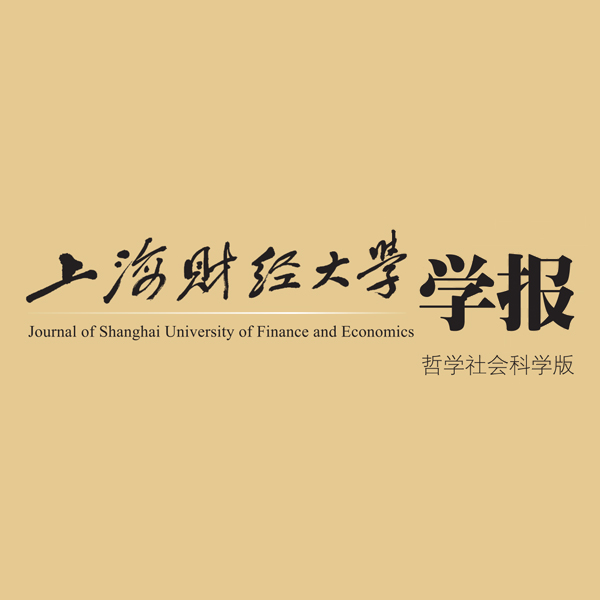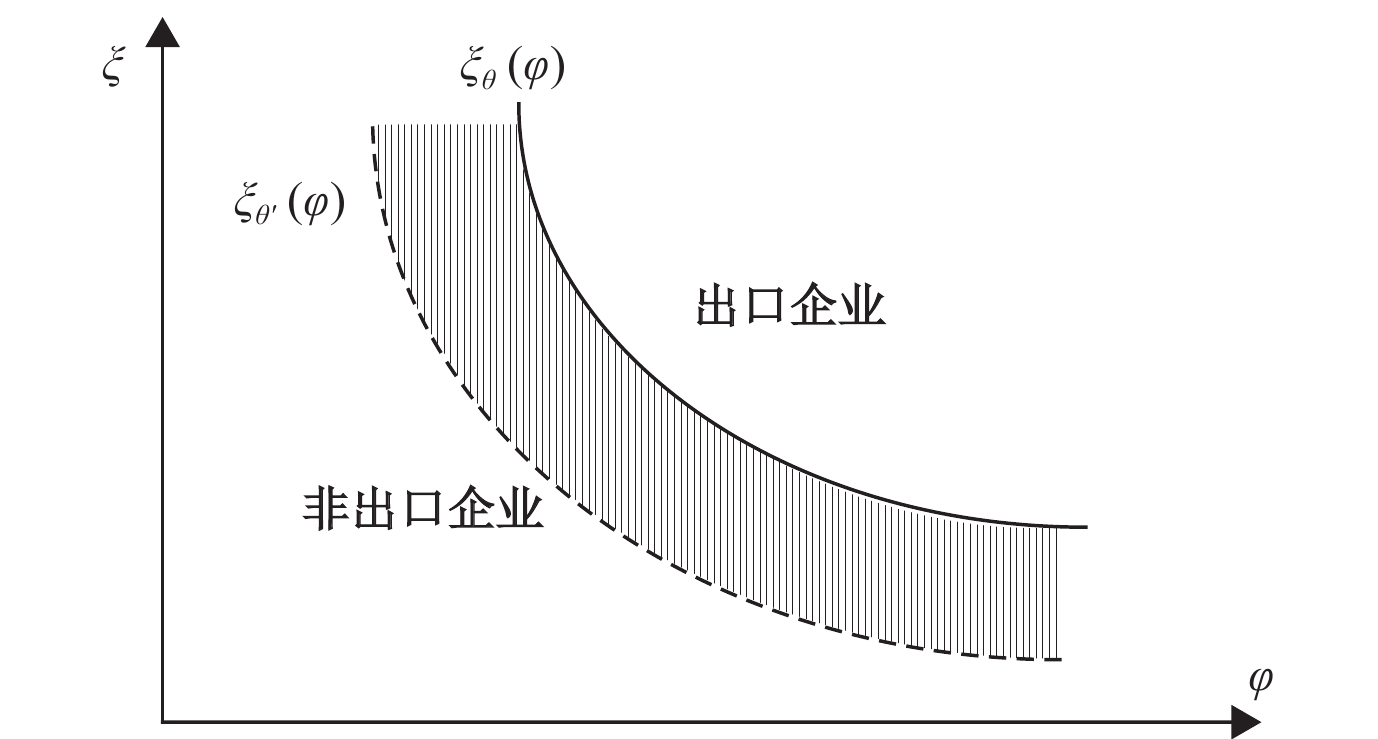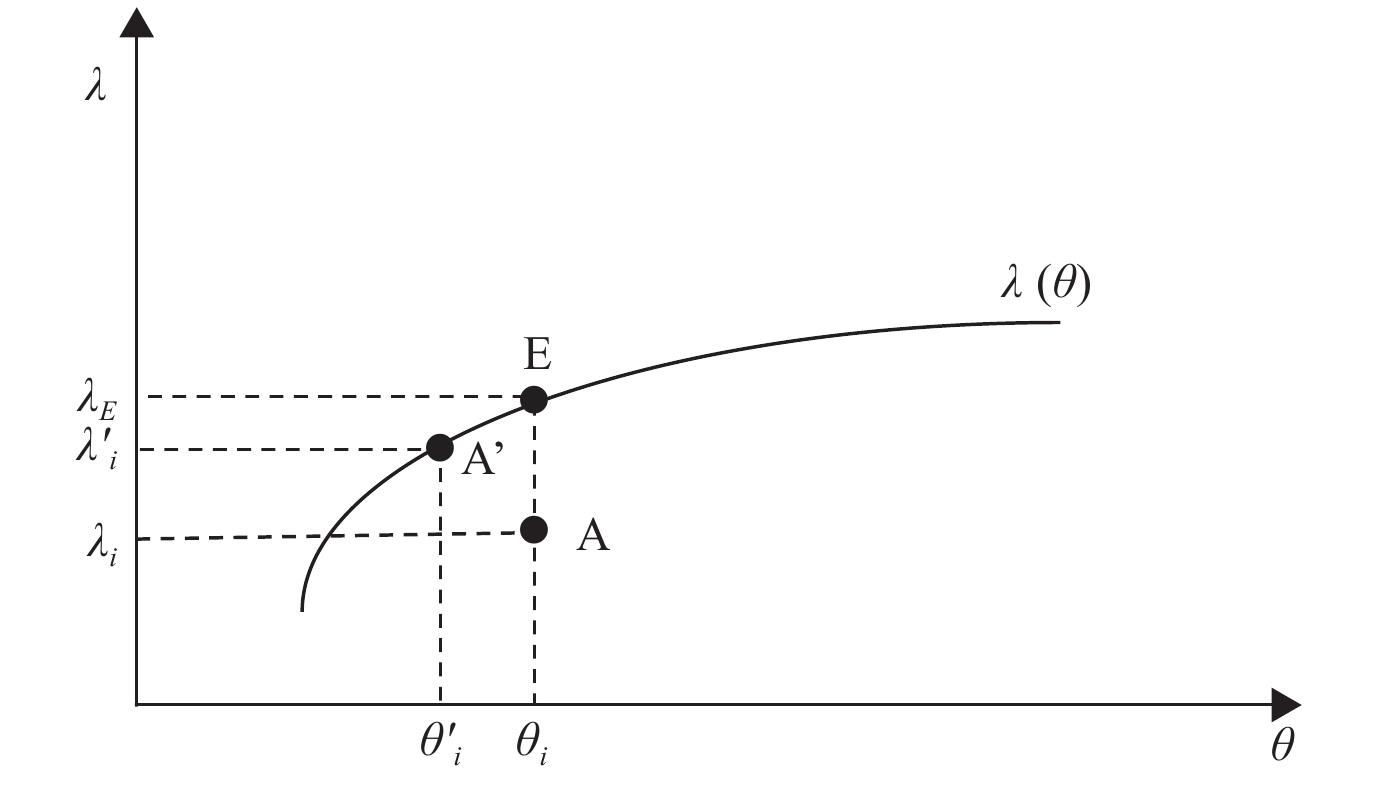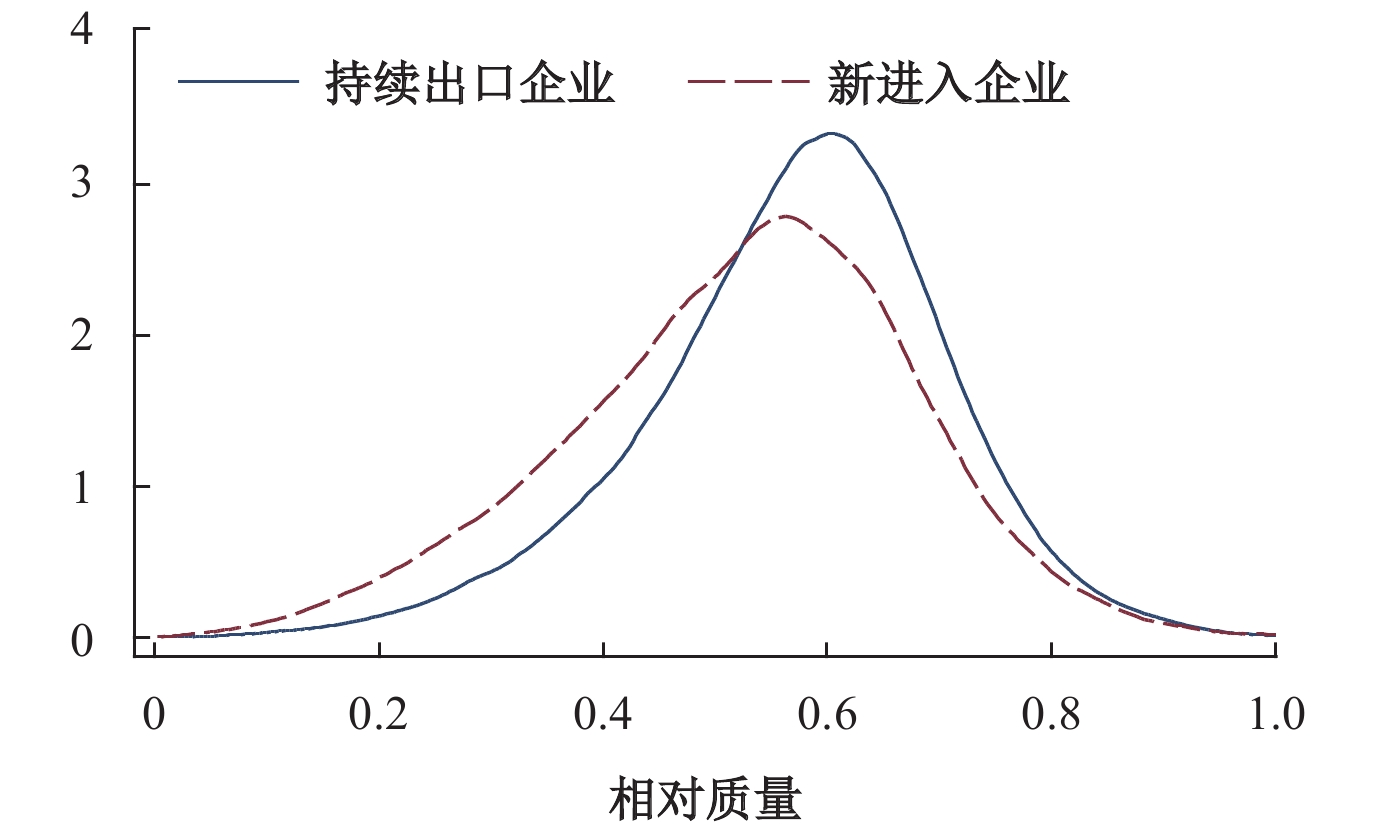﻿ 银行业竞争对中国企业出口质量升级的“双刃剑”效应《上海财经大学学报》
2021第23卷第2期

1.山东财经大学 金融学院，山东 济南 250014;
2.山东财经大学 国际经贸学院，山东 济南 250014

 $U={\left[\sum\nolimits_{imt}{\left({\lambda }_{imt}{q}_{imt}\right)}^{\tfrac{\sigma -1}{\sigma }}\right]}^{\tfrac{\sigma }{\sigma -1}}$ (1)

${\rm{ \mathrm{\lambda }}}$

$\mathrm{q}$

$\mathrm{\sigma }>1$

$\mathrm{i}$
、m和t分别代表企业、出口目的地和时间。由此可得消费者对企业产品的需求式为：

 $q={p}_{}^{-\sigma }{\lambda }_{}^{\sigma -1}\frac{E}{P}$ (2)

$\mathrm{p}$

${\rm{\mathrm{\lambda }}}$

$\mathrm{E}$

$P={\int }_{x}^{}{p}^{1-\sigma }{\lambda }^{\sigma -1}dx$

 $r=pq={\left(\frac{p}{\lambda }\right)}^{1-\sigma }\frac{E}{P}$ (3)

$\mathrm{r}$

$\mathrm{c}$
）与固定成本（
$\mathrm{F}$
）构成，见式（4）。一方面，可变成本与生产率呈反向关系，与产品质量呈正向关系；另一方面，除了企业运营需要支付一部分固定成本（
${\mathrm{F}}_{0}$
）外，生产高质量产品的企业还需支付部分额外固定成本，包括价格更高的机器设备等。

 $c\left(\lambda ,\varphi \right)=\frac{\gamma }{\varphi }{\lambda }^{\beta } ; F\left(\lambda ,\xi \right)={F}_{0}+\frac{f}{\xi }{\lambda }^{a}$ (4)

${\rm{\mathrm{c}}}$
$\mathrm{F}$

${\rm{\mathrm{\gamma }}}$
$\mathrm{f}$

${\mathrm{F}}_{0}$

${\rm{ \mathrm{\varphi }}}$

${\rm{\mathrm{\lambda }}}$

${\rm{\mathrm{\xi }}}$

$0\leqslant \beta < 1$

$a>\left(1-\beta \right)\left(\sigma -1\right)$

$[1-\theta \left(bc\right)]$
，则
$\theta \left(bc\right)F\left(\lambda ,\xi \right)$

$\theta \left(bc\right)$

$\mathrm{b}\mathrm{c}$

$\partial \theta /\partial bc<0$

(二) 银行业竞争下的融资约束与企业最优产品质量的选择

$p=\dfrac{\sigma }{\sigma -1}\dfrac{\gamma }{\varphi }{\lambda }^{\beta }$
，据此，企业出口利润为：

 $\pi =r-cq-\theta \left({F}_{0}+\frac{f}{\xi }{\lambda }^{a}\right)={\left(\frac{\gamma }{\varphi }\right)}^{1-\sigma }{\left(\frac{\sigma }{\sigma -1}\right)}^{-\sigma }{\lambda }^{\left(\beta -1\right)\left(1-\sigma \right)}\frac{1}{\sigma -1}\frac{E}{P}-\theta \left({F}_{0}+\frac{f}{\xi }{\lambda }^{a}\right)$ (5)

 $\lambda ={\left[\frac{1-\beta }{a}{\left(\frac{\sigma -1}{\sigma }\right)}^{\sigma }{\left(\frac{\varphi }{\gamma }\right)}^{\sigma -1}\frac{\xi }{\theta \left(bc\right)f}\frac{E}{P}\right]}^{\tfrac{1}{a-\left(\beta -1\right)\left(1-\sigma \right)}}$ (6)

${\rm{\mathrm{\theta }}}$
）越低，基于利润最大化，企业选择的最优产品质量（
${\rm{\mathrm{\lambda } }}$
）越高。又由于银行业竞争有助于企业缓解融资约束，所以，银行业竞争有利于出口企业产品质量升级。

(三) 银行业竞争下的融资约束与企业出口临界条件

$\pi \geqslant 0$

 $\xi \left(\varphi \right)={\theta }^{\frac{a}{{a}'}}f{\left(\frac{{F}_{0}}{H}\right)}^{\tfrac{a-{a}'}{{a}'}}{\left(\frac{E}{P}\right)}^{\tfrac{-a}{{a-a}'}}{\left(\frac{\varphi }{\gamma }\right)}^{\tfrac{-a\left(\sigma -1\right)}{a-{a}'}}$ (7)

${a}'=a-\left(\beta -1\right)\left(1-\sigma \right)$
$H={\left(\frac{\sigma -1}{\sigma }\right)}^{\tfrac{a\sigma -{a}'}{{a}'}}{\left(\frac{1-\beta }{a}\right)}^{\tfrac{a-{a}'}{{a}'}}$

$\xi$

$\mathrm{\varphi }$

$\mathrm{\theta }$

$\mathrm{\xi }$

${\mathrm{\xi }}_{\mathrm{\theta }}\left(\mathrm{\varphi }\right)$图 1 企业出口临界条件下的生产率与质量生产能力

$\mathrm{\theta }$

${\mathrm{\xi }}_{{\mathrm{\theta }}'}\left(\mathrm{\varphi }\right)$
，导致部分生产率
$\mathrm{\varphi }$

$\xi$

$\xi$

 $\lambda ={{\theta }^{\tfrac{\left(\beta -1\right)\left(1-\sigma \right)}{{\left[\alpha -\left(\beta -1\right)\left(1-\sigma \right)\right]}^{2}}}\left[\frac{1-\beta }{a}{\left(\frac{\sigma -1}{\sigma }\right)}^{\sigma }{\left(\frac{\varphi }{\gamma }\right)}^{\sigma -1}\frac{1}{f}\frac{E}{P}\right]}^{\tfrac{1}{a-\left(\beta -1\right)\left(1-\sigma \right)}}$ (8)

$\mathrm{\theta }$

$\lambda$

$\mathrm{\lambda }\left(\mathrm{\theta }\right)$

$\mathrm{i}$

${\mathrm{\theta }}_{\mathrm{i}}$
，产品质量为
${\mathrm{\lambda }}_{\mathrm{i}}$
，企业位于临界曲线之下，说明在现有融资约束条件下，企业无法进行出口。现假设银行竞争的加剧导致企业面临的融资约束降低至
${\mathrm{\theta }}_{\mathrm{i}}'$
，此时，若质量生产能力不变，根据式（6），在利润最大化下，企业的产品质量提升到
${\mathrm{\lambda }}_{\mathrm{i}}'$
，达到了出口临界条件。基于此，对于企业
$\mathrm{i}$

${\mathrm{\theta }}_{\mathrm{i}}$

${\mathrm{\lambda }}_{\mathrm{E}}$
，企业才能出口；而当融资约束降至
${\mathrm{\theta }}_{\mathrm{i}}'$

${\mathrm{\lambda }}_{\mathrm{i}}'$图 2 银行业竞争下的融资约束与企业出口质量临界条件

(二) 变量选取与描述

1. 被解释变量−企业出口质量水平（

${\rm{{quality}}}_{\rm{{it}}}$

 $ln{q}_{ipmt}={\delta }_{t}-\sigma ln{p}_{ipmt}+\epsilon_{ipmt}$ (9)

$\mathrm{l}\mathrm{n}{\mathrm{q}}_{\mathrm{i}\mathrm{p}\mathrm{m}\mathrm{t}}$

$\mathrm{i}$
$\mathrm{t}$

$\mathrm{m}$

$\mathrm{p}$

$\mathrm{l}\mathrm{n}{\mathrm{p}}_{\mathrm{i}\mathrm{p}\mathrm{m}\mathrm{t}}$

${\delta }_{t}=ln{E}_{t}-ln{P}_{t}$

$\epsilon_{ipmt}=\left(\sigma -1\right)ln{\lambda }_{ipmt}$
，进一步推导出企业出口产品的质量为：

 ${quality}_{ipmt}=ln{\lambda }_{ipmt}=\frac{\epsilon_{ipmt}}{\sigma -1}$ (10)

$\mathrm{i}$
$\mathrm{t}$

$\mathrm{m}$

$\mathrm{p}$

 $s\_{quality}_{ipmt}=\frac{{quality}_{ipmt}-min{quality}_{ipmt}}{max{quality}_{ipmt}-min{quality}_{ipmt}}$ (11)

$\mathrm{m}\mathrm{a}\mathrm{x}$
$\mathrm{m}\mathrm{i}\mathrm{n}$

$\mathrm{s}\_{\mathrm{q}\mathrm{u}\mathrm{a}\mathrm{l}\mathrm{i}\mathrm{t}\mathrm{y}}_{\mathrm{i}\mathrm{p}\mathrm{m}\mathrm{t}}$

$\mathrm{i}$
$\mathrm{t}$

$\mathrm{p}$
$\mathrm{m}$

 ${quality}_{it}=\sum\nolimits_{pm}\frac{{Ex}_{ipmt}}{{\displaystyle\sum }_{pm}{Ex}_{ipmt}}\times s\_{quality}_{ipmt}$ (12)

${\mathrm{q}\mathrm{u}\mathrm{a}\mathrm{l}\mathrm{i}\mathrm{t}\mathrm{y}}_{\mathrm{i}\mathrm{t}}$

$\mathrm{i}$
$\mathrm{t}$

${\mathrm{E}\mathrm{x}}_{\mathrm{i}\mathrm{p}\mathrm{m}\mathrm{t}}$

$\mathrm{i}$
$\mathrm{t}$

$\mathrm{p}$
$\mathrm{m}$

${\displaystyle\sum }_{\mathrm{p}\mathrm{m}}{\mathrm{E}\mathrm{x}}_{\mathrm{i}\mathrm{p}\mathrm{m}\mathrm{t}}$

$\mathrm{i}$
$\mathrm{t}$

2. 解释变量−银行业竞争（

${\rm{Comp}_{ct}}$

${\mathrm{H}\mathrm{H}\mathrm{I}}_{\mathrm{c}\mathrm{t}}$
）（Chong等，2013）。鉴于
${\mathrm{H}\mathrm{H}\mathrm{I}}_{\mathrm{c}\mathrm{t}}$

${Compe}_{ct}=1-{HHI}_{ct}$

 ${{Comp}_{ct}=1-HHI}_{ct}=1-{\sum }_{n=1}^{N}{\left({Branch}_{cnt}/{Branch}_{ctotalt}\right)}^{2}$ (13)

${\mathrm{B}\mathrm{r}\mathrm{a}\mathrm{n}\mathrm{c}\mathrm{h}}_{\mathrm{c}\mathrm{n}\mathrm{t}}$
$\mathrm{t}$

$\mathrm{n}$

$\mathrm{c}$

${\mathrm{B}\mathrm{r}\mathrm{a}\mathrm{n}\mathrm{c}\mathrm{h}}_{\mathrm{c}\mathrm{t}\mathrm{o}\mathrm{t}\mathrm{a}\mathrm{l}\mathrm{t}}$
$\mathrm{t}$
$\mathrm{c}$

3. 控制变量

${\mathrm{l}\mathrm{n}\mathrm{s}\mathrm{i}\mathrm{z}\mathrm{e}}_{\mathrm{i}\mathrm{t}}$
），采用企业销售额的自然对数衡量。（2）企业生产率（
${\mathrm{l}\mathrm{n}\mathrm{t}\mathrm{f}\mathrm{p}}_{\mathrm{i}\mathrm{t}}$
），采用LP方法估算，并以对数形式纳入模型。（3）企业年龄（
$\mathrm{l}\mathrm{n}{\mathrm{a}\mathrm{g}\mathrm{e}}_{\mathrm{i}\mathrm{t}}$
），参照魏浩和李晓庆（2019）、Fan等（2015），采用当期年份减去企业注册年份加1再取自然对数衡量。（4）企业资本密集度（
${\mathrm{l}\mathrm{n}\mathrm{k}\mathrm{l}}_{\mathrm{i}\mathrm{t}}$
），用企业固定资产净值与劳动力人数比值的自然对数衡量。据此，各变量的统计性描述如表1所示。

 变量 变量含义 观测值 均值 标准差 最小值 最大值 ${quality}_{it}$ 企业出口的相对质量 216741 0.5535 0.1433 0 1 ${Comp}_{ct}$ 各地市的银行业竞争度 215733 0.8294 0.0627 0.3091 0.9282 ${lnsize}_{it}$ 企业规模 104748 10.6454 1.3200 0 18.8907 ${lntfp}_{it}$ 企业生产率 104937 −0.0307 0.8341 −11.4394 4.7373 ${lnage}_{it}$ 企业年龄的自然对数 122004 1.9457 0.6727 0 4.0271 ${lnkl}_{it}$ 企业资本/劳动比的自然对数 123455 1.1976 0.4915 −5.9283 2.2918

 2000年 2001年 2002年 2003年 2004年 2005年 2006年 企业出口质量 整体企业 0.4841 0.5053 0.5256 0.5517 0.5451 0.5723 0.5947 持续出口企业 − 0.5132 0.5356 0.5629 0.5561 0.5806 0.6031 新进出口企业 − 0.4462 0.5007 0.5389 0.5373 0.5677 0.5918 银行业竞争程度 0.7983 0.8040 0.8142 0.8223 0.8276 0.8357 0.8542

 ${quality}_{it}={\beta }_{0}+{\beta }_{1}{Compe}_{ct}+{\beta }_{2}{Z}_{it}+{\delta }_{t}+{\delta }_{i}+{\delta }_{d}+{\delta }_{c}+{\epsilon }_{icdt}$ (14)

${\mathrm{quality}}_{\mathrm{it}}$

$\mathrm{i}$
$\mathrm{t}$

${\mathrm{Compe}}_{\mathrm{ct}}$

$\mathrm{i}$

$\mathrm{c}$

${\mathrm{Z}}_{\mathrm{it}}$

${\mathrm{lnsize}}_{\mathrm{it}}$
）、生产率（
${\mathrm{lntfp}}_{\mathrm{it}}$
）、年龄（
$\mathrm{ln}{\mathrm{age}}_{\mathrm{it}}$
）和资产密集度（
${\mathrm{lnkl}}_{\mathrm{it}}$
）；
${\mathrm{\delta }}_{\mathrm{t}}$
${\mathrm{\delta }}_{\mathrm{i}{\text{、}}}{\mathrm{\delta }}_{\mathrm{d}}$
${\mathrm{\delta }}_{\mathrm{c}}$

 （1） （2） （3） （4） （5） （6） （7） （8） （9） （10） ${Comp}_{ct}$ −0.139*** −0.113*** −0.0232** −0.0341** −0.0352*** −0.113*** −0.103*** −0.0318** −0.0375** −0.0403*** （−28.00） （−16.97） （−2.48） （−2.51） （−2.58） （−15.00） （−13.94） （−2.13） （−2.48） （−2.64） ${lnsize}_{it}$ 0.0038*** 0.0057*** 0.0270*** 0.0263*** 0.0263*** （7.54） （11.25） （23.63） （22.75） （22.77） ${lntfp}_{it}$ 0.0116*** 0.0112*** −0.0028** −0.0026** −0.0027** （15.44） （14.76） （−2.20） （−2.09） （−2.10） ${lnage}_{it}$ −0.0046*** −0.0047*** 0.0001 0.0011 0.0011 （−6.61） （−6.86） （0.07） （0.51） （0.50） ${lnkl}_{it}$ −0.0143*** −0.0122*** −0.0048*** −0.0042*** −0.0042*** （−15.10） （−12.02） （−3.49） （−3.10） （−3.09） 常数项 0.669*** 0.652*** 0.573*** 0.588*** 0.589*** 0.639*** 0.608*** 0.303*** 0.313*** 0.315*** （161.66） （117.20） （73.96） （52.12） （51.74） （78.58） （74.85） （17.47） （17.84） （17.91） 时间效应 YES YES YES YES YES YES YES YES YES YES 企业效应 NO NO YES YES YES NO NO YES YES YES 行业效应 NO YES NO YES YES NO YES NO YES YES 地区效应 NO NO NO NO YES NO NO NO NO YES N 215733 124281 196521 108146 108142 100780 100743 85163 85110 85109 R2 0.057 0.144 0.770 0.799 0.799 0.081 0.165 0.807 0.811 0.811 说明：括号内为t统计值，*p < 0.10，**p < 0.05，***p < 0.01，“YES”表明加入了固定效应，下同。
(二) 稳健性检验

1. 内生性问题

${\rm{compeiv}}$
）与该城市银行业竞争程度（
$comp$
）存在显著的正相关性，且F检验值大于经验法则建议的10，表明选取的工具变量是有效的；第二阶段的结果表明，当采用工具变量进行回归时，银行业竞争的系数依然为负，意味着银行业竞争度的加剧在总体上不利于企业出口质量的改善，与前文结果一致。

 工具变量法 替换核心变量 替换控制变量 第一阶段 第二阶段 银行业竞争度 企业出口质量 企业产值 变量平减处理 $comp$ −0.139*** −0.0108* −0.0085** −0.0408*** −0.0403*** （−4.99） （−1.67） （−2.48） （−2.68） （−2.64） $compeiv$ 0.891*** （158.28） CV YES YES YES YES YES YES N 101112 101112 85372 58170 85450 85109 R 0.6053 0.804 0.810 0.813 0.810 0.811 F 23.94*** − − − 注：CV表示与前文基准回归相同的控制变量，并加入了企业、时间、地区和行业固定效应，下同

2. 替换变量

（1）替换银行业竞争度指标。参照姜付秀等（2019），本文采用各地市前三大银行支行数量占银行支行总数的比例（

${\rm{CR3}}$
），作为银行业竞争的替换变量，模型如下：

 $CR3=\left({Branch}_{1th}+{Branch}_{2th}+{Branch}_{3th}\right)/{Branch}_{totalt}$ (15)

${\mathrm{Branch}}_{1\mathrm{th}}$
${\mathrm{Branch}}_{2\mathrm{th}}$
${\mathrm{Branch}}_{3\mathrm{th}}$

$\mathrm{t}$

${\mathrm{Branch}}_{\mathrm{ctotalt}}$
$\mathrm{t}$

$1-{\rm{CR3}}$

（2）替换企业出口质量指标。在企业出口质量的测算上，由于式（9）中的产品质量

${\mathrm{\epsilon}}_{\mathrm{i}\mathrm{p}\mathrm{m}\mathrm{t}}$

${\mathrm{p}}_{\mathrm{i}\mathrm{p}\mathrm{m}\mathrm{t}}$

（3）替换控制变量。本文一方面采用企业产值的自然对数作为规模的替换变量，另一方面考虑到变量跨年度的可比性，对企业规模等变量进行平减处理，结果均见表4。根据结果可知，在替换了控制变量后，银行业竞争变量的系数仍然显著为负，与基准回归结果一致。

(三) 原因解析

1. 融资约束机制的检验

 ${FC}_{it}={\gamma }_{0}+{\gamma }_{1}{Compe}_{ct}+{\gamma }_{2}{Z}_{it}+{\delta }_{t}+{\delta }_{i}+{\delta }_{d}+{\delta }_{c}+{\epsilon }_{icdt}$ (16)
 ${quality}_{it}={\eta }_{0}+{\eta }_{1}{Compe}_{ct}+{\eta }_{2}{FC}_{it}+{\eta }_{3}{Z}_{it}+{\delta }_{t}+{\delta }_{i}+{\delta }_{d}+{\delta }_{c}+{\epsilon }_{icdt}$ (17)

$\mathrm{F}\mathrm{C}$

$FC=\left|SA\right|=\left|0.043{lntasset}^{2}-0.04age-0.737lntasset\right|$
，值越大，表明企业面临的融资约束越强。其中，
${\rm{lntasset}}$

$\mathrm{a}\mathrm{g}\mathrm{e}$

 （1）企业出口质量 （2）融资约束 企业出口质量 新进出口企业 （3）持续出口 （4）新进出口 （5）出口质量 （6）银行业竞争 ${Comp}_{ct}$ −0.0403*** −0.0604*** −0.0166 −0.164*** −0.0496*** −0.0402** （−2.64） （−3.66） （−0.62） （−5.39） （−3.20） （−2.54） ${FC}_{it}$ −0.0162*** 0.00713** （−6.23） （2.51） ${entry}_{it}$ −0.0256*** 0.00932 （−24.25） （0.73） ${Comp}_{ct}\times {entry}_{it}$ −0.0425*** （−2.76） $CV$ YES YES YES YES YES YES N 85109 85109 64745 10742 78081 78081 R2 0.811 0.979 0.821 0.801 0.824 0.824

2. 新进出口企业的质量分析

 $\begin{split} {quality}_{it}=&{\beta }_{0}+{\beta }_{1}{comp}_{ct}+{\beta }_{2}{entry}_{it}+{\beta }_{3}{comp}_{ct}\times {entry}_{it}+{\beta }_{4}{Z}_{it}+ \\ &{\delta }_{t}+{\delta }_{i}+{\delta }_{d}+{\delta }_{c}+{\epsilon }_{icdt} \end{split}$ (18)

${\mathrm{entry}}_{\mathrm{it}}$

${\text{β}}_{3}$
，若该系数显著为负，则意味着银行业竞争的加剧导致新进企业出口质量的下降，回归结果见表5。第一，在第（5）列中，新进出口企业变量（
${\mathrm{entry}}_{\mathrm{it}}$
）为负，说明新进企业出口质量偏低，与前文典型化事实描述一致。同时，利用图3描绘持续出口企业与新进出口企业的产品质量分布，可以看出持续出口企业的平均质量显著高于新进出口企业。第二，本文加入新进出口企业虚拟变量与银行业竞争交互项（
${\mathrm{Comp}}_{\mathrm{ct}}\times$
${\mathrm{entry}}_{\mathrm{it}}$
），结果见第（6）列，交互项系数显著为负，意味着银行业竞争程度越高，新进出口企业的产品质量越低，即银行业竞争在一定程度上降低了该地区新进出口企业的质量水平，这可能是因为银行业竞争的加剧导致银行在贷款和监管力度上的放松，总体上缓解了企业的融资约束，降低了企业出口的质量门槛，使一些低质量企业进入出口市场，拉低了出口企业的平均质量水平。图 3 持续出口企业与新进出口企业的产品质量水平对比

 ${statebankc}_{ct} = {\sum }_{n=1}^{{N}_{s}}{\left({Branch}_{cnt}/{Branch}_{ctotalt}\right)}^{2}/HHI$ (19)
 ${stockbankc}_{ct} = {\sum }_{n=1}^{{N}_{j}}{\left({Branch}_{cnt}/{Branch}_{ctotalt}\right)}^{2}/HHI$ (20)
 ${citybankc}_{ct} = {\sum }_{n=1}^{{N}_{c}}{\left({Branch}_{cnt}/{Branch}_{ctotalt}\right)}^{2}/HHI$ (21)
 ${forbankc}_{ct} = {\sum }_{n=1}^{{N}_{f}}{\left({Branch}_{cnt}/{Branch}_{ctotalt}\right)}^{2}/HHI$ (22)

${\mathrm{N}}_{\mathrm{s}}$
${\mathrm{N}}_{\mathrm{j}}$
${\mathrm{N}}_{\mathrm{c}}$
${\mathrm{N}}_{\mathrm{f}}$

 （1）国有银行 （2）城市银行 （3）股份制银行 （4）外资银行 ${Comp}_{ct}$ −0.0590**（−2.42） −0.0392**（−2.56） 0.0155（0.63） −0.0461***（−2.85） ${statebankc}_{ct}$ −0.0110（−1.22） ${Comp}_{ct}\times {statebankc}_{ct}$ 0.161*（1.66） ${citybankc}_{ct}$ 0.0153**（2.04） ${Comp}_{ct}\times {citybankc}_{ct}$ 0.00636（0.07） ${stockbankc}_{ct}$ −0.176***（−4.29） ${Comp}_{ct}\times {stockbankc}_{ct}$ 3.002**（2.35） ${forbankc}_{ct}$ −10.64（−0.26） ${Comp}_{ct}\times {forbankc}_{ct}$ −358.9（−1.14） $CV$ YES YES YES YES N 70919 85109 85109 85109 R2 0.812 0.811 0.811 0.811 注：为防止交互项的多重共线性问题，对各变量进行了中心化处理。
(二) 股份制改革的影响

2003年我国实施股份制商业银行改革，开始推进建立现代金融企业制度，据此，本文以2003年为界进行分组检验，结果如表7所示。结果显示，股份制改革以前，银行业竞争的系数显著为负，意味着银行业竞争的加剧会显著抑制中国企业出口质量的升级；但在股份制改革以后，银行业竞争的系数变为正值且不显著，说明银行业竞争对我国企业出口质量的抑制作用不再明显。我们将银行业竞争的二次项变量（

${\rm{{Comp}_{ct}}^{2}}$
）纳入模型，见表7第（2）列和第（4）列。结果显示，股改前，银行业竞争对企业出口质量的非线性影响并不显著；但股改后，银行业竞争系数显著为正，且二次项系数显著为负。这说明股份制改革后，银行业竞争对企业出口质量呈现倒U形影响，即在竞争不充分时，银行业竞争加剧虽会显著促进企业出口质量升级，但随着竞争程度的进一步白热化，该正向影响逐渐减弱。同时，银行业竞争导致低资质企业进入的负面影响逐步增大，进而对企业出口质量呈现出抑制作用。

 （1）股份制改革前 （2）股份制改革后 （3）股份制改革前 （4）股份制改革后 ${Comp}_{ct}$ 0.0707*** −0.210* 0.0377 1.118** （−3.79） （−1.69） （0.65） （2.23） ${{Comp}_{ct}}^{2}$ 0.0899 −0.684** （1.14） （-2.17） $CV$ YES YES YES YES N 22186 22186 56606 56606 R2 0.840 0.840 0.853 0.853

(三) 基于企业所有权类型的分组检验

 国有企业 私营企业 全样本 股份制改革前 股份制改革后 全样本 股份制改革前 股份制改革后 ${Comp}_{ct}$ 0.0381 0.232 0.230* −0.0820*** −0.236* −0.0646** （0.45） （1.29） （1.72） （−2.95） （−1.70） （−2.32） $CV$ YES YES YES YES YES YES N 4064 2033 1636 13542 2502 9574 R2 0.808 0.820 0.881 0.824 0.836 0.887

① 方便起见，以下公式省去下标。

② 采用2000–2006年数据的原因为：一是此时期涵盖国有银行股份制改革前后，也是我国建立现代银行制度的关键期，对此分析不仅能反映不同时期我国银行业竞争对出口质量的影响，还能为我国银行业改革的经济效果研究提供经验证据；二是2007年后微观变量缺失严重，为保证结果准确性，仅保留2000–2006年数据。

③ 若企业上一期出口为0，当期出口大于0，则将该企业认定为新进出口企业；若企业上一期和当期出口均大于0，则将该企业认定为持续出口企业。

④ 由于部分企业只存在一个出口市场，故利用工具变量法对出口质量进行处理，会损失一定的样本量。

  边文龙, 沈艳, 沈明高. 银行业竞争度、政策激励与中小企业贷款−来自14省90县金融机构的证据[J].金融研究,2017(1).  蔡竞, 董艳. 银行业竞争与企业创新−来自中国工业企业的经验证据[J].金融研究,2016(11).  蔡卫星. 银行业市场结构对企业生产率的影响−来自工业企业的经验证据[J].金融研究,2019(4).  陈雄兵. 银行竞争、市场力量与货币政策信贷传导[J].财贸经济,2017(2).  姜付秀, 蔡文婧, 蔡欣妮, 等. 银行竞争的微观效应: 来自融资约束的经验证据[J].经济研究,2019(6).  施炳展. 中国企业出口产品质量异质性: 测度与事实[J].经济学（季刊）,2013(1).  施炳展, 邵文波. 中国企业出口产品质量测算及其决定因素−培育出口竞争新优势的微观视角[J].管理世界,2014(9).  魏浩, 李晓庆. 知识产权保护与中国企业进口产品质量[J].世界经济,2019(6).  张杰, 郑文平, 翟福昕. 中国出口产品质量得到提升了么?[J].经济研究,2014(10).  张杰. 金融抑制、融资约束与出口产品质量[J].金融研究,2015(6).  张璇, 李子健, 李春涛. 银行业竞争、融资约束与企业创新−中国工业企业的经验证据[J].金融研究,2019(10).  Allen F, Qian J, Qian M J. Law, finance, and economic growth in China[J].Journal of Financial Economics,2005,77(1):57–116.  Beck T, Demirguc-Kunt A, Maksimovic V. Bank competition and access to finance: International evidence[J]. Journal of Money, Credit, and Banking,2004,36(3):627–648.  Bernini M, Guillou S, Bellone F. Firms leverage and export quality evidence from France[R]. Working Papers hal-00973035, HAL, 2013.  Brandt L, Van Biesebroeck J, Zhang Y F. Creative accounting or creative destruction? Firm-level productivity growth in Chinese manufacturing[J].Journal of Development Economics,2012,97(2):339–351.  Broecker T. Credit-worthiness tests and interbank competition[J].Econometrica,1990,58(2):429–452.  Chaney T. Liquidity constrained exporters[J]. Journal of Economic Dynamics and Control,2016,72:141–154.  Chen Y L, Liu M, Su J. Greasing the wheels of bank lending: Evidence from private firms in China[J]. Journal of Banking & Finance,2013,37(7):2533–2545.  Chong T T L, Lu L P, Ongena S. Does banking competition alleviate or worsen credit constraints faced by small-and medium-sized enterprises? Evidence from China[J]. Journal of Banking & Finance,2013,37(9):3412–3424.  Cornaggia J, Mao Y F, Tian X, et al. Does banking competition affect innovation?[J]. Journal of Financial Economics,2015,115(1):189–209.  Crinò R, Ogliari L. Financial imperfections, product quality, and international trade[J]. Journal of International Economics,2017,104:63–84.  Fan H C, Lai E L C, Li Y A. Credit constraints, quality, and export prices: Theory and evidence from China[J]. Journal of Comparative Economics,2015,43(2):390–416.  Hallak J C, Sivadasan J. Product and process productivity: Implications for quality choice and conditional exporter Premia[J]. Journal of International Economics,2013,91(1):53–67.  Khandelwal A. The long and short（of）quality ladders[J]. The Review of Economic Studies,2010,77(4):1450–1476.  Krugler M, Verhoogen E. Prices, plants size, and product quality[J].Review of Economics Studies,2012,79(1):307–339.  Love I, Martínez P, Maria S. How bank competition affects firms’ access to finance[J].World Bank Economic Review,2014,29(3):413–448.  Manova K. Credit constraints, heterogeneous firms, and international trade[J].The Review of Economic Studies,2013,80(2):711–744.  Melitz M J. The impact of trade on intra-industry reallocations and aggregate industry productivity[J].Econometrica,2003,71(6):1695–1725.  Petersen M A, Rajan R G. The effect of credit market competition on lending relationships[J].Quarterly Journal of Economics,1995,110(2):407–443.  Zarutskie R. Evidence on the effects of bank competition on firm borrowing and investment[J]. Journal of Financial Economics,2006,81(3):503–537.
“Double-edged Sword” Effect of Banking Competition on the Export Quality Upgrading of Chinese Enterprises：Based on Dual Perspectives of Quality Adjustment and Export Entry
Liu Hui1, Wang Mingyi2
1.School of Finance，Shandong University of Finance and Economics，Shandong Jinan 250014，China;
2.School of International Economics and Trade，Shandong University of Finance and Economics，Shandong Jinan 250014，China